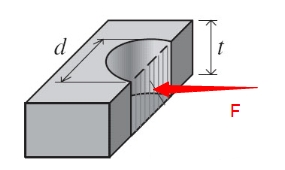This is the bearing stress calculator to find the stress around the hole on the plates.F (lbs) = d (in)= t (in)= t is the thickness of the plate.d is the diameter of the hole.F is the applied load on the bolt.